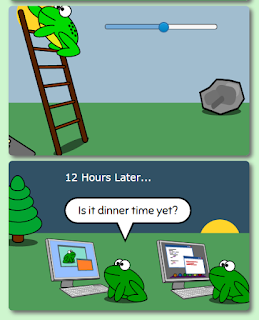Monday, February 1, 2016The comic frames with the color gradient backgrounds.
For the comic on Friday, I had to generate a color gradient between the full-brightness daylight color in the third frame and the nighttime darkness color in the fourth frame. Since the point of the comic was to adjust the brightness using a slider with 20 positions, I needed 40 colors (including the 2 starting colors) so that when the brightness was all the way down both frames would have the same background. They would meet in the middle.

It was actually a very nice comic. On the right you can see the frames with the color gradients, but the real comic is interactive - go play around with it if you haven't yet!

To generate the gradient of colors, I found a set of JavaScript functions which I will share here. I can't take credit for it, all I did was rearrange some of the code and re-order the output (the original seemed backwards to me) but it is very nice. I am keeping it in the source code for the Amphibian.com comic editor in case I ever need it again.

Here is the function. Calling it should be self-explanatory. Note that the colorCount parameter will be the total size of the output array INCLUDING the start and end colors.

```function generateColorGradient(colorStart, colorEnd, colorCount) {

/* Convert a hex string to an RGB triplet */
var convertToRGB = function(hex) {

/* Remove '#' in color hex string */
var trim = function(s) {
return (s.charAt(0) == '#') ? s.substring(1, 7) : s;
};

var color = [];
color = parseInt((trim(hex)).substring(0, 2), 16);
color = parseInt((trim(hex)).substring(2, 4), 16);
color = parseInt((trim(hex)).substring(4, 6), 16);
return color;

};

/* Convert an RGB triplet to a hex string */
var convertToHex = function(rgb) {

var hex = function(c) {
var s = "0123456789abcdef";
var i = parseInt(c);
if (i == 0 || isNaN(c))
return "00";
i = Math.round(Math.min(Math.max(0, i), 255));
return s.charAt((i - i % 16) / 16) + s.charAt(i % 16);
};

return hex(rgb) + hex(rgb) + hex(rgb);

};

var start = convertToRGB(colorStart);
var end = convertToRGB(colorEnd);

// The number of colors to compute
var len = colorCount - 1;

//Alpha blending amount
var alpha = 0.0;

var ret = [];
ret.push(convertToHex(start));

for (i = 0; i < len; i++) {
var c = [];
alpha += (1.0 / len);
c = end * alpha + (1 - alpha) * start;
c = end * alpha + (1 - alpha) * start;
c = end * alpha + (1 - alpha) * start;
ret.push(convertToHex(c));
}

ret[len] = convertToHex(end); // last color might be slightly off

return ret;

}
```

One thing that I noticed is that for colorCount values greater than 13 the final color was not quite the requested final color. Rounding error I suppose. That's why I explicitly set the last element in the return array to the end color, if you were wondering.

Using jQuery, I wrote a simple demonstration of the gradient function:

```    var things = generateColorGradient("#CC0033", "#FFFF66", 15);
for (var i = 0; i < things.length; i++) {
\$("body").append("<div style='background-color: #" + things[i] + ";'>test</div>");
}

```

...which produces the following output on a web page. Beautiful, isn't it?

Today's comic, on the other hand, contains absolutely nothing of any technical interest. You should still read it though. And if you haven't read all the other Amphibian.com comics, what are you waiting for? They don't take that long to read. Start today!Amphibian.com comic for 1 February 2016